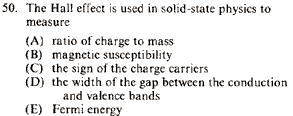GR 8677927796770177 | # Login | Register

GR8677 #50
Problem
 GREPhysics.NET Official Solution Alternate SolutionsAdvanced Topics}Solid State Physics

Recall , where the sign shows that the charge carrier is negative. ( is the electron density, electron charge, speed of light)

The Hall effect has to do with a bar of metal placed in a magnetic field. An electric current runs through it perpendicular to the field direction. However, the magnetic field term in the Lorentz force creates a force perpendicular to both the magnetic field and electric field. This pulls" the current astride from its original straight path. Charge starts accumulating on one side of the slab from this force, even as current continues to flow perpendicular to the accumulation direction. In equilibrium, the magnetic force balances the electric force from this charge accumulation. Apply some basic EM theory, and one can derive the Hall coefficient () defined above.Alternate Solutions
 There are no Alternate Solutions for this problem. Be the first to post one!
resinoth
2015-09-17 21:20:48
\"The Hall effect is a conduction phenomenon which is different for different charge carriers. In most common electrical applications, the conventional current is used partly because it makes no difference whether you consider positive or negative charge to be moving. But the Hall voltage has a different polarity for positive and negative charge carriers, and it has been used to study the details of conduction in semiconductors and other materials which show a combination of negative and positive charge carriers.\"\r\nfrom hyperphysicsjondiced
2010-10-18 18:15:07
The Hall effect is basically when electrical charges get pulled to the side of a conductor because of a magnetic field. The Lorentz force from the magnetic field depends on the sign of the charge carrier, so oppositely-charged particles will go in different directions.

When they migrate they create an electric field perpendicular to the direction of the current, and the direction of the field tells you the sign of the charge carriers.Albert
2009-10-17 09:00:23
I do not think it is C.

One can refer to the link below. The Hall effect tells not the sign of the charge carrier but the carrier density as well as the magnetic field. I may be wrong, I am not too sure but Wikipedia suggests otherwise.

http://en.wikipedia.org/wiki/Hall_effect#Quantum_Hall_effect
 hdcase2009-10-25 21:26:33 The answer is C because if you were to stick an unknown semiconductor in a magnetic field with a perpendicular voltage you could measure the charge accumulation (in the direction perpendicular to both the magnetic field and the electrical field) to determine whether holes or electrons were moving along the direction of the applied current.
 rhasi2010-07-25 09:51:28 does it depend on interger or fractional quantum hall effects?
 pam d2011-09-24 11:34:42 This question is classical in nature.aaa
2007-07-14 13:54:32
 aaa2007-07-14 13:55:34 c is the answerYou are replying to:
The Hall effect is basically when electrical charges get pulled to the side of a conductor because of a magnetic field. The Lorentz force from the magnetic field depends on the sign of the charge carrier, so oppositely-charged particles will go in different directions. When they migrate they create an electric field perpendicular to the direction of the current, and the direction of the field tells you the sign of the charge carriers.LaTeX syntax supported through dollar sign wrappers $, ex.,$\alpha^2_0$produces . type this... to get...$\int_0^\infty\partial\Rightarrow\ddot{x},\dot{x}\sqrt{z}\langle my \rangle\left( abacadabra \right)_{me}\vec{E}\frac{a}{b}\$